# Scale-Sensitive Fractal Analysis

## To mark the release of the new Scale-Sensitive Fractal Analysis (SSFA) optional module, now available to Mountains® software users, here is an introduction on what SSFA is all about and how to use it.

### 1. What is fractal analysis?

We all learned at school that geometrical objects are sorted by the number of coordinates required to describe them. A line segment is 1-dimensional, a plane is 2-dimensional and a cube is 3-dimensional. However, in the real world, lines may be more complicated than that.

Benoit Mandelbrot, the American-French-Polish mathematician, described the basis of a new branch of mathematics where geometrical dimensions could be fractional instead of integer. This theory had many interesting applications in physics, economy and even the arts. The fractal dimension was found to be an interesting parameter to describe physical surfaces, and especially engineering surfaces.A rough surface is like a microscopic mountainous surface, and its fractal dimension can be seen as an attribute characterizing its complexity. A very smooth and planar surface will have a fractal dimension close to 2.0 but a ceramic surface, which features many pores and cavities, will have a higher value, maybe closer to 3.0 than 2.0.

The work of Mandelbrot applied to engineering surfaces was developed in particular by Professor Christopher Brown of Worcester Polytechnic Institute (WPI) who introduced length-scale and area-scale analyses, together with several other parameters and statistical methods to establish functional correlations and discriminations.

These techniques are designated by the acronym SSFA – Scale-Sensitive Fractal Analysis and are also sometimes referred to as Geometric Multiscale Analysis.

### 2. Scale-Sensitive graphs

When using length-scale analysis, a line-segment of fixed length (the reference scale) is used to measure the actual length of a profile (or a surface line). The cumulated length divided by the horizontal length is called relative length. This value is between 1.0 and 2.0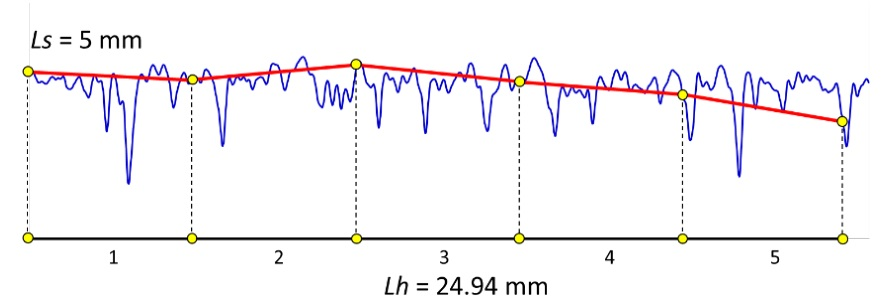In the example above a segment of 5 mm length is used to measure a profile. We can fit 5 segments into a horizontal length of 24.94 mm. The relative length is therefore 25.0/24.94 = 1.0024.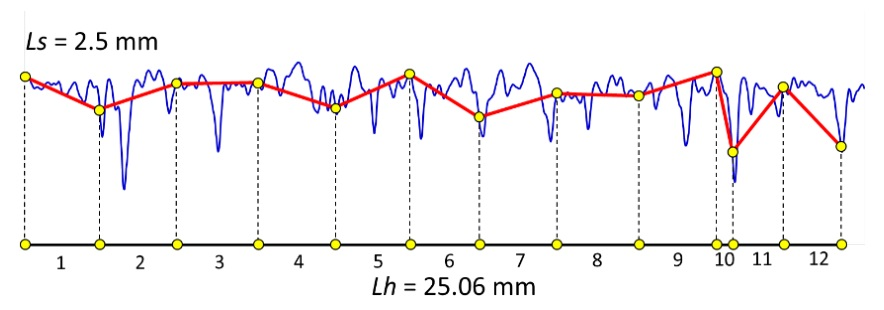However, if we use a segment of 2.5 mm it is possible to fit 12 segments into a horizontal length of 25.06 mm, which gives a relative length of 1.197. In other words, the smaller the segment, the longer the measured length and the higher the relative length.

The measure is repeated with different segment lengths and a graph is drawn: log(relative length) in function of log(scale).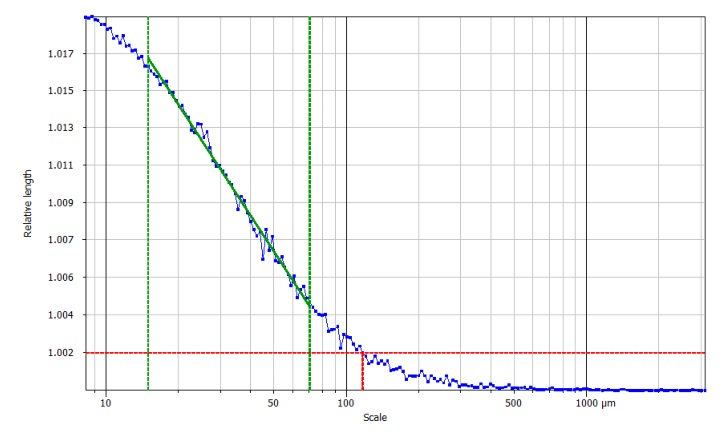On this graph a threshold is set to find the smooth-rough crossover (SRC) which gives the scale of transition between large scales where the surface is analyzed from too far away (its fractal dimension is close to its Euclidian dimension) and scales where the fractal dimension really does describe the behavior of the surface. The central part, between the two green lines, is almost linear and its slope is used to calculate the fractal dimension.

### 3. Can a surface be analyzed using the same method?

The answer to this is yes, since relative length can be calculated on lines or on columns of the surface and the average value of relative length used to build the graph.

Alternatively, area-scale method can be used, where triangular tiles of a fixed area are used to calculate the actual area of the surface, and the relative area is obtained by division with the projected area. A similar graph is obtained and the same parameters can be calculated.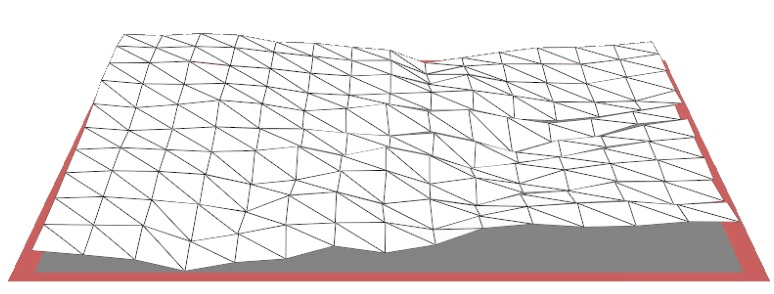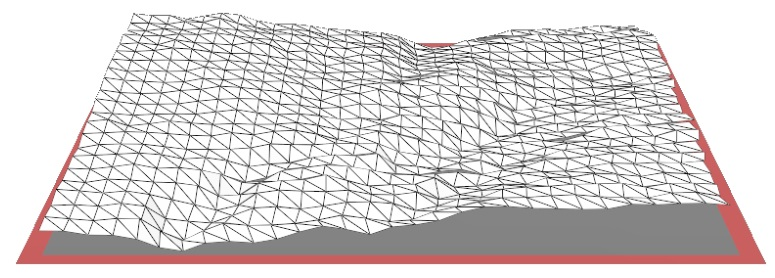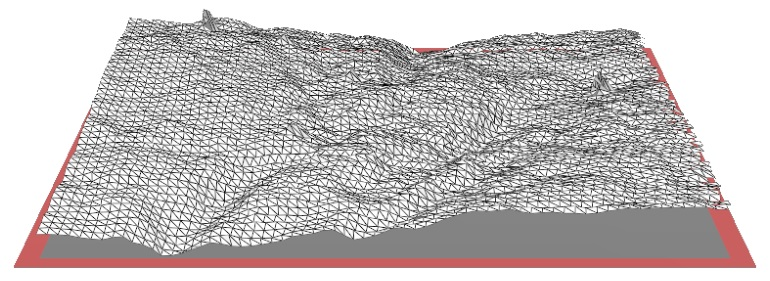Above. Three tiling exercises with different tile sizes.

### 4. Related parameters

These parameters are used in various fields of metrology and research such as the study of adhesion strength, fracture analysis, thermal-spray coating, tool wear etc.

Smooth-rough crossover: this parameter is very useful for applications related to friction and contact.

Maximum relative length/area: the value at the finest scale. On a surface, this value converges towards the ISO parameter Sdr.

Fractal dimension: calculated on the linear part of the graph.

Complexity: characterizes how much the surface is more complex than a Euclidian plane (or a profile from a Euclidian line). This concept is somewhat similar to the ISO parameter Sdq.

Scale of maximum complexity: scale at which the surface exhibits its higher complexity. This parameter can be used in correlation studies with functional attributes.

Regression coefficient R²: a high value confirms the accuracy of the complexity value.

Heterogeneity of complexity: where the surface is analyzed by blocks (for example 5x5) and the dispersion of complexity is calculated.

Plus some parameters initially developed for Dental Microwear Texture Analysis by Prof. Rob Scott and Prof. Peter Ungar.

One advantage is that these parameters are calculated within a scale range where the function is found to be active. This scale-limited approach is more powerful than calculating a field parameter on a surface with a default filter.

See our Surface Metrology Guide videos on scale sensitive approaches:

The parameters listed above are described in ISO 25178-2:2021, clause 4.7 and are also part of ASME B46.1:2002.

### 5. Where can I find the tools?

MountainsMap® offers an optional module called Scale-Sensitive Fractal Analysis module which implements most of the methods that were available in Sfrax software (developed by Prof. C. Brown). This module can be tested for free for 30 days and purchased as a separate module.